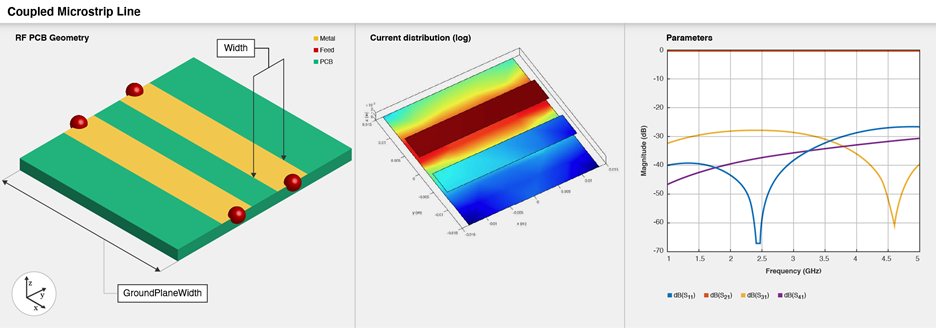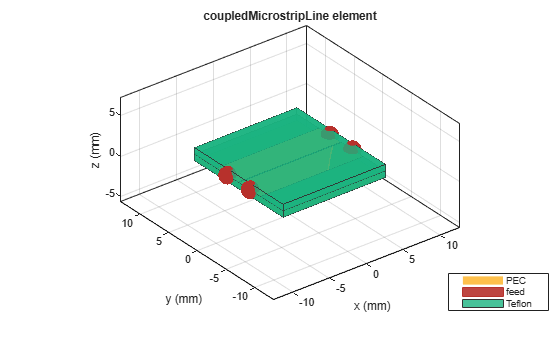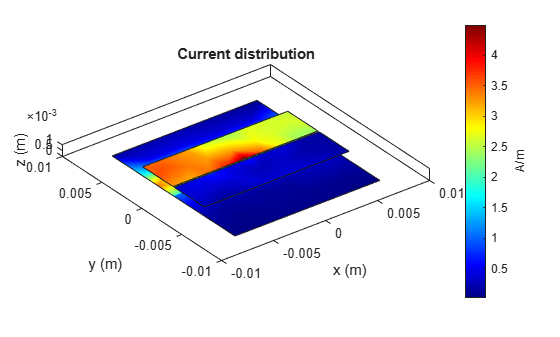# coupledMicrostripLine

Create coupled microstrip transmission line

Since R2021b

## Description

Use the `coupledMicrostripLine` object to create a coupled microstrip transmission line. Coupled microstrip transmission lines are used to design directional couplers and filters. The combination of even and odd mode impedances determines the coupling ratio between the direct arm and the coupled arm.To analyze the behavioral model for the coupled microstrip line, set the `Behavioral` property in the `sparameters` to `true` or 1.

## Creation

### Syntax

``coupledmicrostrip = coupledMicrostripLine``
``coupledmicrostrip = coupledMicrostripLine(Name=Value)``

### Description

example

````coupledmicrostrip = coupledMicrostripLine` creates a default coupled microstrip transmission line with a Teflon substrate and default properties for a resonating frequency of 1.5 GHz.```

example

````coupledmicrostrip = coupledMicrostripLine(Name=Value)` sets Properties using one or more name-value arguments. For example, `coupledMicrostripLine(Length=0.0300)` creates a coupled microstrip transmission line of length 0.0300 meters.```

## Properties

expand all

Length of the coupled microstrip line in meters, specified as a positive scalar.

Example: ```coupledmicrostrip = coupledMicrostripLine(Length=0.0300)```

Data Types: `double`

Width of the coupled microstrip line in meters, specified as a positive scalar.

Example: ```coupledmicrostrip = coupledMicrostripLine(Width=0.0041)```

Data Types: `double`

Distance between the direct arm and the coupled arm of the coupled microstrip transmission line, specified as a positive scalar in meters.

Example: ```coupledmicrostrip = coupledMicrostripLine(Spacing=0.00300)```

Data Types: `double`

Height of the coupled microstrip line from the ground plane, specified as a positive scalar in meters. In the case of a multilayer substrate, use the `Height` property to create a coupled microstrip line at the interface of the two dielectrics.

Example: ```coupledmicrostrip = coupledMicrostripLine(Height=0.0023)```

Data Types: `double`

Width of the ground plane in meters, specified as a positive scalar.

Example: ```coupledmicrostrip = coupledMicrostripLine(GroundPlaneWidth=0.0400)```

Data Types: `double`

Type of the dielectric material used as a substrate, specified as a `dielectric` object. The dielectric material in a `coupledMicrostripLine` object with default properties is Teflon. The thickness of the default dielectric material Teflon is `0.0016` m or the same as the `Height` property.

Example: `d = dielectric("FR4");` `coupledmicrostrip = coupledMicrostripLine(Substrate=d)`

Data Types: `string` | `char`

Type of metal used in the conducting layers, specified as a `metal` object. The type of metal in a `coupledMicrostripLine` object with default properties is PEC.

Example: `m = metal("PEC");` `coupledmicrostrip =coupledMicrostripLine(Conductor=m)`

Data Types: `string` | `char`

Flag to add a metal shielding to the PCB component, specified as a logical 0 or logical 1.

Example: `IsShielded = true` or `1` add a metal shield.

Note

To enable FEM solver required for the metal shield property, download the `Integro-Differential Modeling Framework for MATLAB`. To download this add-on:

1. In the Home tab Environment section, click on . This opens the add-on explorer. You need an active internet connection to download the add-on.

2. Search for ```Integro-Differential Modeling Framework for MATLAB``` and click .

`matlab.addons.installedAddons`
in your MATLAB® session command line.

Data Types: `logical`

Metal shield for the PCB component, specified as a `shape.Box` object. The length and width of the box must be equal to the length and width of the ground plane. The center of the box is at `[0 0 Height]`. You can modify the property after creating the object.

#### Dependencies

To enable the `Shielding` property, set the `IsShielded` property to `true` or `1`.

Type of RF connector assembled at the feed locations of the PCB component, specified as a `RFConnector` object.

Example: Create connector from `RFConnector` object like this: `coaxial = RFConnector` adds a coaxial connector.

#### Dependencies

To enable the `Connector` property, set the `IsShielded` property to `true` or `1`.

## Object Functions

 `charge` Calculate and plot charge distribution `current` Calculate and plot current distribution `design` Design coupled microstrip transmission line around particular frequency `feedCurrent` Calculate current at feed port `getZ0` Calculate characteristic impedance of transmission line `layout` Plot all metal layers and board shape `mesh` Change and view mesh properties of metal or dielectric in PCB component `shapes` Extract all metal layer shapes of PCB component `show` Display PCB component structure or PCB shape `sparameters` Calculate S-parameters for RF PCB objects `RFConnector` Create RF connector

## Examples

collapse all

Create a default coupled microstrip line.

`cml = coupledMicrostripLine`
```cml = coupledMicrostripLine with properties: Length: 0.0271 Width: 0.0051 Spacing: 0.0046 Height: 0.0016 GroundPlaneWidth: 0.0300 Substrate: [1x1 dielectric] Conductor: [1x1 metal] IsShielded: 0 ```

View the coupled microstrip line.

`show(cml)`Design and view a coupled microstrip line at the interface of a multilayer dielectric.

```cml = design(coupledMicrostripLine,4e9,Z0e=75,Z0o=36); cml.Substrate = dielectric(Name=["Teflon","Teflon"],EpsilonR=[2.1 2.1], ... LossTangent=[0 0],Thickness=[0.8e-3 0.8e-3]); cml.Height = 0.8e-3; show(cml)```Plot the current and charge distribution on the transmission line.

`current(cml,4e9)````figure charge(cml,4e9)```